# GSEB Solutions Class 7 Maths Chapter 5 Lines and Angles InText Questions

Gujarat Board GSEB Textbook Solutions Class 7 Maths Chapter 5 Lines and Angles InText Questions and Answers.

## Gujarat Board Textbook Solutions Class 7 Maths Chapter 5 Lines and Angles InText QuestionsTry These (Page 94)

Question 1.
List ten figures around you and identify the acute, obtuse and right angles found in them.
Solution:
It is an activity. Please do it yourself.

Think, Discuss and write (Page 95)

Question 1.
Can two acute angles be complement to each other?
Solution:
Yes.
For example, 30° is an acute angle and 60° is also an acute angle.
Since, 30° + 60° = 90°
∴ 30° and 60° are complementary angles, i.e. two acute angles can be complement to each other.

Question 2.
Can two obtuse angles be complement to each other?
Solution:
No.
An obtuse angle has its measure greater than 90°. Therefore, sum of two obtuse angles cannot be equal to 90°, i.e. they cannot be complement to each other.Question 3.
Can two right angles be complement to each other?
Solution:
No.
1 right angle = 90°
Therefore, two right angles cannot be complement to each other.

Try These (page 95)

Question 1.
Which pairs of following angles are complementary?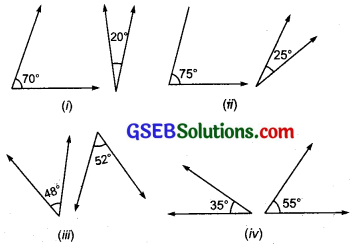(i) Since 70° + 20° = 90°
∴ Angles 70° and 20° are complementary.

(ii) ∵ 75° + 25° = 100° and 100° ≠ 90°
∴ Angles 75° and 25° are not complementary.

(iii) ∵ 48° + 52° = 100° and 100° ≠ 90°
∴ The angles 48° and 52° are not complementary.

(iv) ∵ 35° + 55° = 90°
∴ The angles 35° and 55° are complementary.Question 2.
What is the measure of the complement of each of the following angles?
(i) 45°
(ii) 65°
(iii) 41°
(iv) 54°
Solution:
(i) Let the complement of 45° be x.
∴ x + 45° = 90° or x = 90° – 45° = 45°
∴ Complement of 45° is 45°.

(ii) Let the complement of 65° be p.
∴ p + 65° = 90° or p = 90° – 65° = 25°
∴ The complement of 65° is 25°.

(iii) Let the complement of 41° be m.
∴ m + 41° = 90°
or m = 90° – 41°
or m = 49°
Thus, the complement of 41° is 49°.

(iv) Let the complement of 54° be y.
∴ y + 54° = 90° or y = 90° – 54° = 36°
Thus, the complement of 54° is 36°.

Question 3.
The difference in the measures of two complementary angles is 12°. Find the measures of the angles.
Solution:
Let one of the angles be x.
∵ Their difference = 12°
∴ The other angle = x + 12°
Since, the sum of the measures of two complementary angles is 90°.
∴ x + (x + 12°) = 90°
or 2x = 90° – 12°
or 2x = 78°
Dividing both sides by 2, we have
$$\frac { 2x }{ 2 }$$ = $$\frac { 78° }{ 2 }$$ or x = 39°
∴ One angle =39°
And the other angle = 39° + 12° = 51°Think, Discuss and Write (Page 96)

Question 1.
Can two obtuse angles be supplementary?
Solution:
No.
The measure of an obtuse angle is more than 90°.
The sum of two angles, (each more than 90°), is more than 180°.
Thus, two obtuse angles cannot be supplementary.

Question 2.
Can two acute angles be supplementary?
Solution:
No.
The measure of an acute angle is less than 90°.
Therefore, the sum of two acute angles is less than 180°.
∴ Two acute angles cannot be supplementary.Question 3.
Can two right angles be supplementary?
Solution:
Yes.
The measure of a right angle is 90°.
∴ The sum of two right angles = 180°
i.e. the two right angles are supplementary.

Try These (Page 96)

Question 1.
Find the pairs of supplementary angles in the following figures: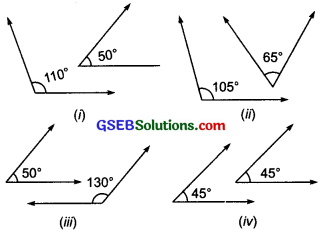Solution:
(i) Measures of the given angles are 110° and 50°.
∵ 110° + 50° = 160° and 160° ≠ 180°
∴ 110° and 50° are not a pair of supplementary angles.

(ii) Measures of the given angles are 105° and 65°.
∵ 105° + 65° = 170° and 170° ≠ 180°
∴ 105° and 65° are not a pair of supplementary angles.

(iii) Measures of the given angles are 50° and 130°.
∵ 50° + 130° = 180°
∴ 50° and 130° are a pair of supplementary angles.

(iv) Measures of the given angles are 45° and 45°.
∵ 45° + 45° = 90° and 90° ≠ 180°
∴ 45° and 45° are not a pair of supplementary angles.Question 2.
What will be the measure of the supplement of each one of the following angles?
(i) 100°
(ii) 90°
(iii) 55°
(iv) 125°
Solution:
(i) Let the supplement of 100° be x.
∴ 100° + x = 180°
or x =180° – 100° = 80°
The measure of the supplement of 100° is 80°.

(ii) Let the supplement of 90° be x.
∴ x + 90° = 180°
or x =180° – 90° = 90°
∴ The measure of the supplement of 90° is 90°.

(iii) Let the supplement of 55° be m.
∴ 55° + m = 180°
or m = 180° – 55°
or m = 125°
∴ The supplement of 55° is 125°.

(iv) Let the supplement of 125° be y.
∴ y + 125° = 180°
or y = 180° – 125°
or y = 55°
∴ The supplement of 125° is 55°.

Question 3.
Among two supplementary angles the measure of the larger angle is 44° more than the measure of the smaller. Find their measures.
Solution:
Let the smaller angle be x.
∴ The measure of the larger angle = (x + 44°)
Since, the two angles are supplementary
∴ x + (x + 44°) = 180°
or 2x + 44° =180°
or 2x = 180° – 44°
or 2x = 136°
or x = $$\frac { 136° }{ 2 }$$
∴ The smaller angle = 68°
Larger angle = 68° + 44° = 112°Try These (Page 97)

Question 1.
Are the angles marked 1 and 2 adjacent? If they are not adjacent, say, ‘why’.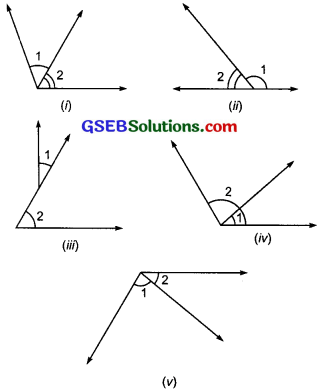Solution:
(i) Yes, ∠1 and ∠2 are adjacent angles.
(ii) Yes, ∠1 and ∠2 are adjacent angles.
(iii) No, ∠1 and ∠2 are not adjacent angles because they have no common vertex.
(iv) No, ∠1 and ∠2 are not adjacent angles because ∠1 is a part of ∠2.
(v) Yes, ∠1 and ∠2 are adjacent angles.

Question 2.
In the given figure, are the following adjacent angles?(a) ∠AOB and ∠BOC
Solution:
(a) Yes, ∠AOB and ∠BOC are adjacent angles, because they have common vertex O and their outer arms (OA and OC) are on either side
of the common arm OB.
(b) No, because ∠BOC is a part of ∠BOD.

Think, Discuss and Write (Page 98)

Question 1.
Can two adjacent angles be supplementary?
Solution: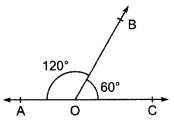Also, ∠AOB + ∠BOC = 180°
∴ ∠AOB and ∠BOC are supplementary.Question 2.
Can two adjacent angles be complementary?
Solution:
Yes, in the figure, ∠PQR and ∠RQS are adjacent angles,
Also ∠PQR + ∠RQS = 90°
i.e. ∠PQR and ∠RQS are complementary angles.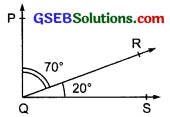Question 3.
Can two obtuse angles be adjacent angles?
Solution:
Yes, in the figure, ∠BOC and ∠COD are obtuse angles and they are adjacent angles.Question 4.
Can an acute angle be adjacent to an obtuse angle?
Solution:
Yes, in the figure, ∠1 and ∠2 are adjacent angles. ∠1 is acute and ∠2 is an obtuse angle.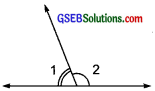Think, Discuss and Write (Page 99)

Question 1.
Can two acute angles form a linear pair?
Solution:
No. The sum of the measures of two acute angles is less than 180° because measures of each acute angle is less than 90°.Question 2.
Can two obtuse angles form a linear pair?
Solution:
No. The sum of the measures of two obtuse angles is more than 180°.

Question 3.
Can two right angles form a linear pair?
Solution:
Yes, because the sum of two right angles is 180°.

Try These (Page 99)

Question 1.
Check which of the following pairs of angles form a linear pair.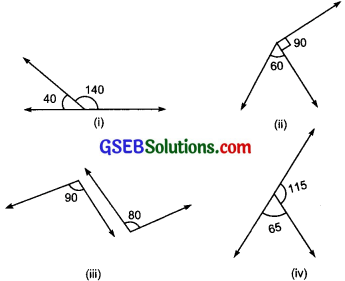Solution:
(i) Yes.
∵ 140° + 40° = 180°
∴ The given pair of angles forms a linear pair.

(ii) No.
∵ 60° + 90° = 150° and 150° ≠ 180°
∴ The given pair of angles does not form a linear pair.

(iii) No.
∵ 90° + 80° = 170° and 170° ≠ 180°
∴ The given pair of angles does not form a linear pair.

(iv) Yes.
∵ 115° + 65° = 180°
∴ The given pair of angles forms a linear pair.Try These (Page 101)

Question 1.
In the given figure, if ∠1 = 30°, find ∠2 and ∠3.
Solution:∵ ∠3 and ∠1 are vertically opposite angles,
∠3 = ∠1
But ∠1 = 30° [Given]
∴ ∠3 = 30°
Again, ∠3 and ∠2 form a linear pair.
∴ ∠3 + ∠2 = 180° or 30° + ∠2 = 180°
or ∠2 = 180° – 30° = 150°
Thus, ∠2 = 150°
and ∠3 = 30°

Question 2.
Give an example for vertically opposite angles in your surroundings.
Solution:Think, Discuss and Write (Page 104)

Question 1.
In the figure, AC and BE intersect at P. AC and BC intersect at C, AC and EC intersect at C. Try to find another ten pairs of intersecting line segments.Solution:
Various pairs of intersecting lines are:
(i) Intersecting at B are:
[CB and EB], [PB and CB]

(ii) Intersecting at C are:
[BC and EC], [PC and BC], [PC and EC] ‘

(iii) Intersecting at E are:
[BE and CE], [PE and CE]

(iv) Intersecting at P are:
[BP and CP], [EP and CP],

(v) [AP and BP] and [AP and EP]

Question 2.
Can two lines intersect in more than one point? Think about it.
Solution:
No, two lines cannot intersect in more than one point.Try These (Page 104)

Question 1.
Find examples from your surroundings where lines intersect at right angles.
Solution:

Question 2.
Find the measure of the angles made by the intersecting lines at the vertices of an equilateral triangle.
Solution: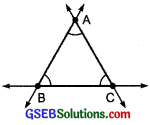Points of intersection are A, B and C.
Measure of ∠A = 60°
Measure of ∠B = 60°
Measure of ∠C = 60°

Question 3.
Draw any rectangle and find the measures of angles at the four vertices made by the intersecting lines.
Solution: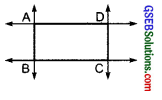Measure of ∠A = 90°
Measure of ∠B = 90°
Measure of ∠C = 90°
Measure of ∠D = 90°

Question 4.
If two lines intersect, do they always intersect at right angles?
Solution:
No.Try These (Page 105)

Question 1.
Suppose two lines are given. How many transversals can you draw for these lines?
Solution:
We can draw an infinite number of transversals to two given lines.

Question 2.
If a line is a transversal to three lines, how many points of intersections are there?
Solution:
As shown in the adjoining figure, there are 3 distinct points of intersection.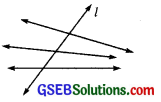Question 3.
Try to identify a few transversals in your surroundings.
Solution:

Try These (Page 106)

Question 1.
Name the pairs of angles in each figure:Solution:
In the figures,
(i) ∠1 and ∠2 are a pair of corresponding angles.
(ii) ∠3 and ∠4 are a pair of alternate interior angles.
(iii) ∠5 and ∠6 are a pair of interior angles on the same side of the transversal.
(iv) ∠7 and ∠8 are a pair of corresponding angles.
(v) ∠9 and ∠10 are a pair of alternate interior angles.
(vi) ∠11 and ∠12 are linear pair of angles.Try These (Page 109)

Question 1.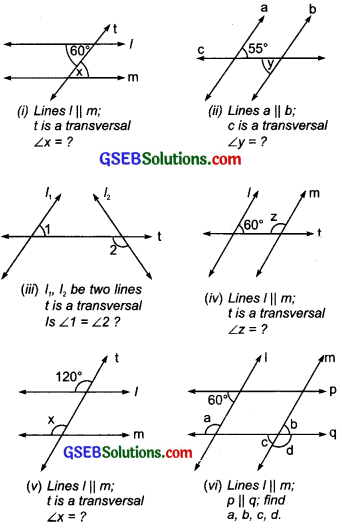Solution:
(i) x = 60°
[∵ x and 60° are alternate interior angles]

(ii) y = 55°
[∵ y and 55° are alternate interior angles]

(iii) No, ∠1 and ∠2 are not equal.
[∵ l1 and 2 are not parallel]

(iv) ∵ 60° + z = 180° ⇒ z = 180° – 60° = 120°
[∵ Z and 60° are interior angles on the same side of the transversal]
∴ z = 120°

(v) x = 120°
[x and 120° are corresponding angles]

(vi) a + 60° = 180°
⇒ a = 180° – 60° = 120°
a = d = 120° [alternate exterior angles]
b + d = 180° [linear pair]
⇒ b + 120° = 180°
⇒ 6 = 180° – 120° = 60°
⇒ c = b = 60°. [vertically opp. angles.]

Try These (Page 110)

Question 1.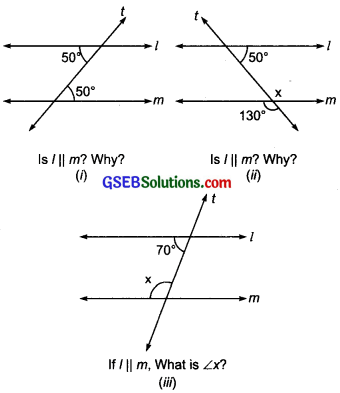Solution:
(i) If a transversal intersects two given lines such that alternate angles are equal, then the given lines are parallel.
∵ 50° = 50°
∵ Alternate angles are equal. Thus, l || m

(ii) l || m as pair of interior angles on the same side of the transversal are supplementary. Here, x = 130° [vertically opposite]
and 50° = 130° = 180° [Supplementary angles]

(iii) ∵  l and m are parallel and t is a transversal,
∴ The sum of interior angles on the same side of the transversal is 180°.
or x + 70° = 180°
or x = 180° – 70°
or x = 110°